Courses

# Important Formulae: Progressions CAT Notes | EduRev

## Quant : Important Formulae: Progressions CAT Notes | EduRev

The document Important Formulae: Progressions CAT Notes | EduRev is a part of the Quant Course Quantitative Aptitude (Quant).
All you need of Quant at this link: Quant

Arithmetic Progression

an = a1 + (n - 1)d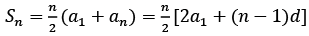EduRev's Tip:

• Number of terms =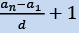Geometric Progression
an = arn - 1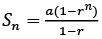Sum till infinite terms =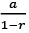(Valid only when r<1)
Sum of first n natural numbers
⇒ 1 + 2 + 3 … + n =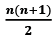Sum of squares of first n natural numbers
⇒ 12 + 22 + 32 + … + n2 =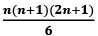Sum of cubes of first n natural numbers

⇒ 13 + 23 + 33 ... + n3 =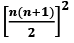• Sum of first n odd numbers
⇒ 1 + 3 + 5 … + (2n - 1) = n2

• Sum of first n even numbers
⇒ 2 + 4 + 6 ... 2n = n(n - 1)

• If you have to consider 3 terms in an AP, consider {a-d,a,a+d}. If you have to consider 4 terms, consider {a-3d,a-d,a+d,a+3d}

• If all terms of an AP are multiplied with k or divided with k, the resultant series will also be an AP with the common difference dk or d/k respectively.

Offer running on EduRev: Apply code STAYHOME200 to get INR 200 off on our premium plan EduRev Infinity!

## Quantitative Aptitude (Quant)

116 videos|131 docs|131 tests

,

,

,

,

,

,

,

,

,

,

,

,

,

,

,

,

,

,

,

,

,

;